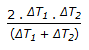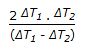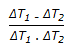# Chemical Engineering - Heat Transfer

### Exercise :: Heat Transfer - Section 7

1.

Intermittant tube cleaning is possible to be done in case of a __________ evaporator.

 A. basket type B. horizontal tube C. calendria D. none of these

Explanation:

No answer description available for this question. Let us discuss.

2.

Harmonic mean temperature difference is given by

 A. ΔT1 . ΔT2 B.C.D.Explanation:

No answer description available for this question. Let us discuss.

3.

Arithmetic mean area can be used in heat transfer problem to calculate the heat flow by conduction through a cylinder which is

 A. thin walled having the value of Ao Ai/< 2. B. thick walled. C. having the value of Ao/Ai > 2. D. both (b) and (c).

Explanation:

No answer description available for this question. Let us discuss.

4.

Three solid objects of the same material and of equal mass-a sphere, a cylinder (length = diameter) and a cube are at 500°C initially. These are dropped in a quenching bath containing a large volume of cooling oil each attaining the bath temperature eventually. The time required for 90% change in temperature is the smallest for

 A. cube B. cylinder C. sphere D. equal for all the three

Explanation:

No answer description available for this question. Let us discuss.

5.

Overall heat transfer co-efficient for cooling of hydrocarbons by water is about

 A. 50 -100 Kcal/hr.m2.°C B. 50 -100 W/m2 . °K C. 50 -100 BTU/hr. ft.2°F D. 1000 - 1500 BTU/hr. ft.2°F